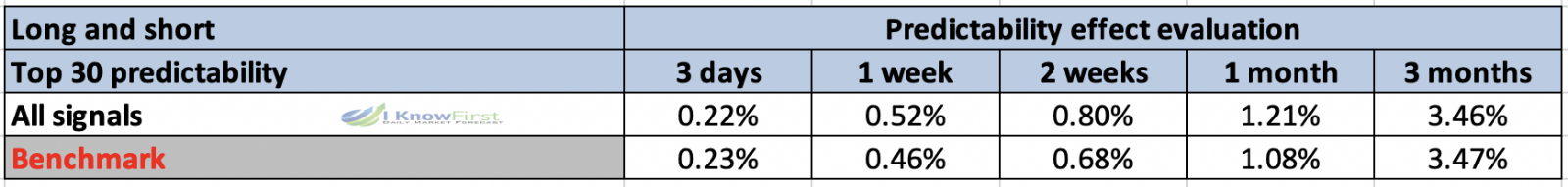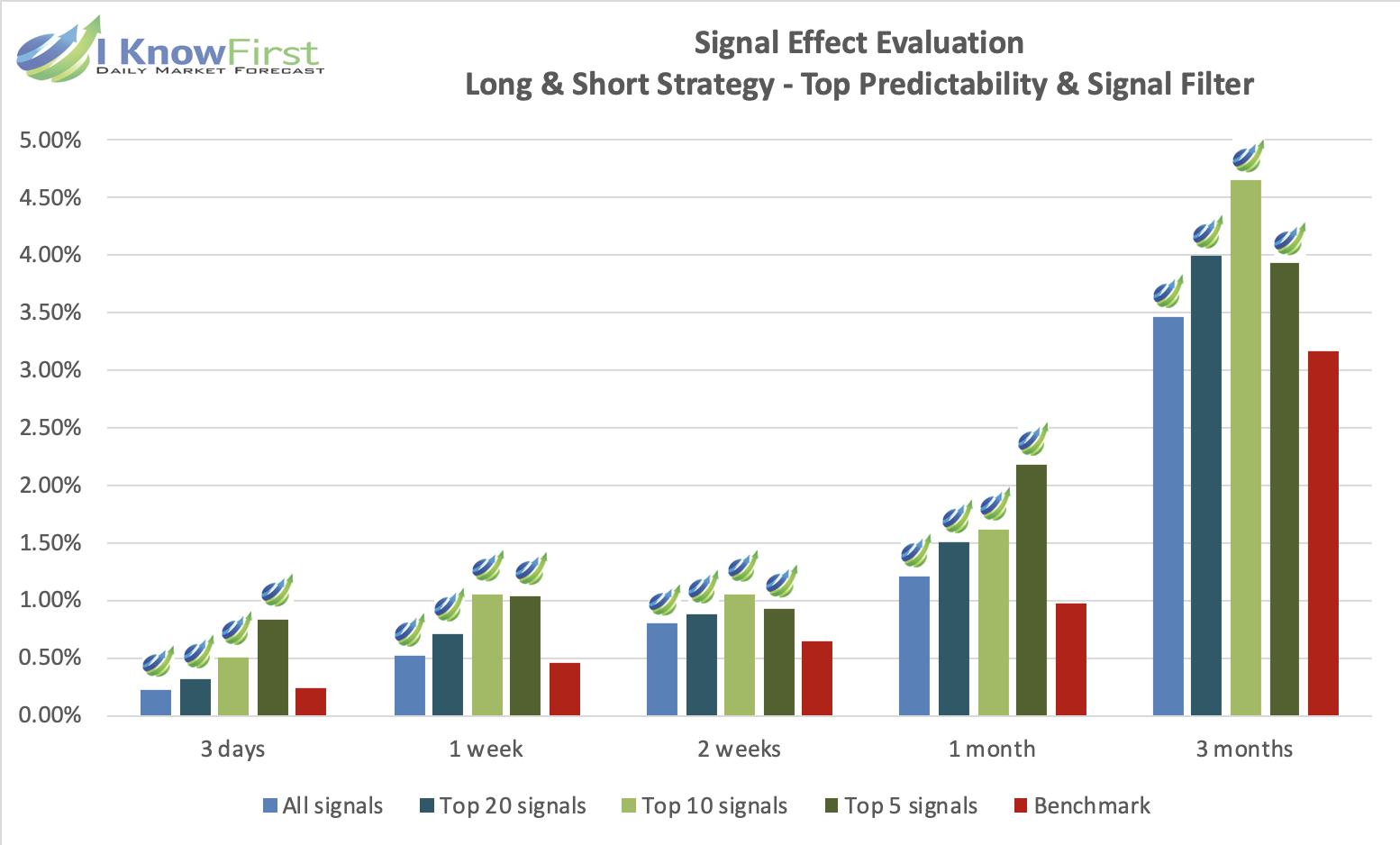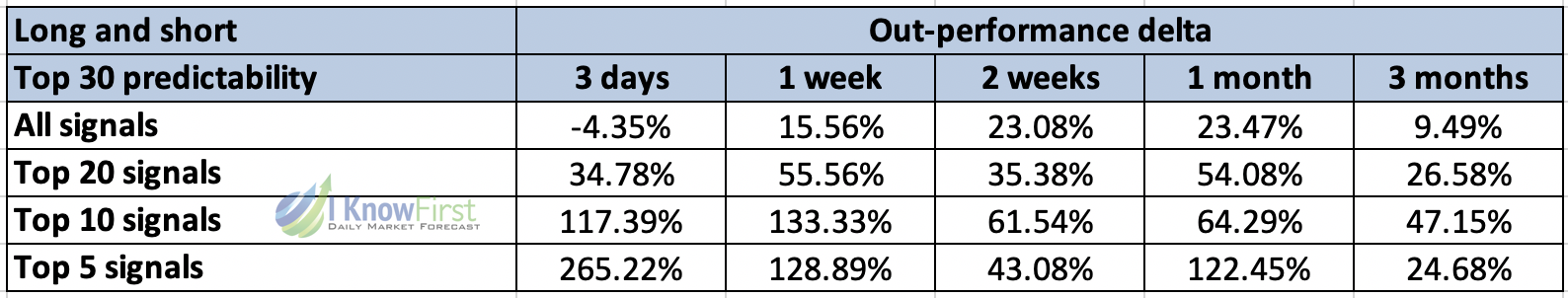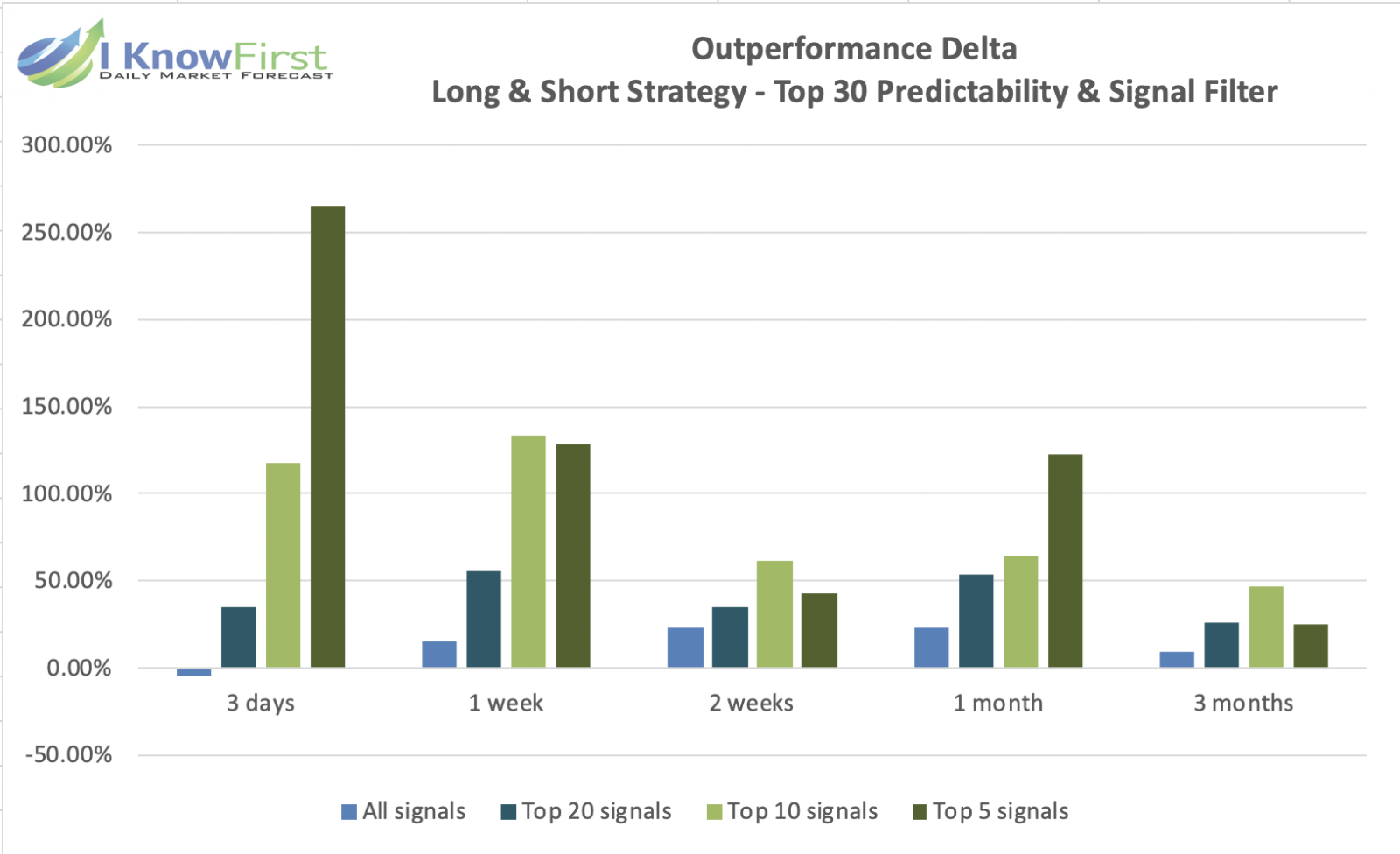# I Know First Evaluation Report for UK Stocks Package

## Executive Summary

In this forecast evaluation report, we will examine the performance of the forecasts generated by the I Know First AI Algorithm for short-term and long-term stocks from the London Stock Exchange which is sent to our customers on a daily basis. Our analysis covers the time period from 1 January 2019 to 30 June 2019. We will start with an introduction to our asset picking and benchmarking methods and then apply it to the UK stock universe as covered by the I Know First’s “UK Stocks” package. We will then compare returns based on our algorithm with the benchmark performance over the same period. Below we present our key takeaways:

### UK Stocks Highlights:

• The best returns were obtained in the 3 months time horizon, with the best performance being a return of 4.65% for the top 10 predictability and signal filtered assets during this time-horizon.
• The top 5 predictability and signal filtered assets beat the benchmark in all time horizons, with the best outperformance being for the 3 day time horizon, where the top 5 assets beat the benchmark by over 265%.

Note that the above results were obtained as a result of evaluation conducted over the specific time period to give a general presentation of the forecast performance patterns for UK stocks. The following report provides extensive explanation on our methodology and detailed analysis of the performance metrics that we obtained during the evaluation. This report is a new I Know First evaluation series illustrating the ability to provide successful long term and flexible forecasting for UK stocks.

## About the I Know First Algorithm

The I Know First self-learning algorithm analyzes, models, and predicts the capital market, including stocks, bonds, currencies, commodities and interest rates markets. The algorithm is based on Artificial Intelligence (AI) and Machine Learning (ML), and incorporates elements of Artificial Neural Networks and Genetic Algorithms.

The system outputs the predicted trend as a number, positive or negative, along with a wave chart that predicts how the waves will overlap the trend. This helps the trader to decide which direction to trade, at what point to enter the trade, and when to exit. Since the model is 100% empirical, the results are based only on factual data, thereby avoiding any biases or emotions that may accompany human derived assumptions. The human factor is only involved in building the mathematical framework and providing the initial set of inputs and outputs to the system. The algorithm produces a forecast with a signal and a predictability indicator. The signal is the number in the middle of the box. The predictability is the number at the bottom of the box. At the top, a specific asset is identified. This format is consistent across all predictions.

Our algorithm provides two independent indicators for each asset – signal and predictability.

The signal is the predicted strength and direction of movement of the asset. This is measured from -inf to +inf.

The predictability indicates our confidence in that result. It is a Pearson correlation coefficient between past algorithmic performance and actual market movement. This is measured from -1 to 1.

You can find a detailed description of our heatmap here.

## The Asset Picking Method

The method in this evaluation is as follows:

To fully utilise information provided by our forecast, we filter out the top X most predictable assets and rank them according to their predictability value. Thereafter, from them, we pick the top Y highest signals and re-adjust the rankings accordingly.

By doing so we focus on the most predictable assets on the one hand, while capturing the ones with the highest signal on the other.

For example, a top 30 predictability filter with a top 10 signal filter means that on each day we take only the 30 most predictable assets from our asset universe, and then we pick from them the top 10 assets with the highest absolute signals. On the other hand, a top 30 predictability filter with a top 30 signal filter would imply that we are solely filtering based on predictability, since we are selecting all assets in this particular set which have already been filtered by predictability.

We use absolute signals since these strategies are long and short ones. If the signal is positive, then we buy assets, i.e. open long position and, if negative, we open short position on such asset. This is to help us to identify the assets with the maximum magnitude of change, which is indiscriminate as to whether one adopts a short or long position.

## The Performance Evaluation Method

We perform evaluations on the individual forecast level. This means that we calculate the return of each forecast we have issued for each horizon in the testing period. We then take the average of those results based on our positions on different assets and forecast horizon.

For example, to evaluate the performance of our 1-month forecasts, we calculate the return of each trade by using this formula:

This simulates a client purchasing the asset on the day we issue our prediction and selling it exactly 1 month in the future from that day.

We iterate this calculation for all trading days in the analyzed period and average the results.

Note that this evaluation does not take a set portfolio and follow it. This is a different evaluation method at the individual forecast level.

## The Hit Ratio Calculation

The hit ratio helps us to identify the accuracy of our algorithm’s predictions.

Using our asset filtering method based on predictability and signal, we predict the direction of movement of different assets. Our predictions are then compared against actual movements of these assets within the same time horizon.

The hit ratio is then calculated as follows:

Hit Ratio (%) = Number of assets correctly predictedTotal number of predicted assets 100

For instance, a 90% hit ratio for a top 30 predictability filter with a top 10 signal filter would imply that the algorithm correctly predicted the price movements of 9 out of 10 assets within this particular set of assets.

## The Benchmarking Method

We utilise two benchmarks to determine the effectiveness of our methodology. An internal benchmark is used to determine the effectiveness of filtering assets based solely on predictability. The FTSE 250 index is used as a benchmark only after filtering assets based on predictability and signal.

In the first case, the theory behind this benchmarking method is the “Null hypothesis“. This means buying every asset in the particular asset universe regardless of our I Know First indicators. For instance, if we were to identify the top 20 stock from a stock universe of 50 stocks, we would calculate the rate of return of 50 stocks, where an equal amount of each stock would be bought at the start of the time horizon and sold at the end of the time horizon. This helps us to determine the effectiveness of our predictability-based asset filtering process by comparing the rate of returns of the benchmark with the rate of return of our predictability-based strategy.

In the second case, particular stocks should be bought (or shorted) when they have been identified to have high signal strength and high predictability. We compare our rate of return based on purchasing (or shorting) the top X assets after applying both the predictability and signal filters with the rate of return of the FTSE 250 index in the same time horizon. This helps us to determine the effectiveness of our methodology against the average investor returns.

The out-performance ratio of our trading results (based on our indicators) to benchmark results indicates the quality of the system and our indicators and provides a measure of competitive advantage an investor could get by using our forecasting solution.

## Asset Universe Under Consideration: UK Stocks

In this report, we conduct testing for 200 stocks trading on the London Stock Exchange that I Know First covers in its algorithmic forecast in the UK Stocks package

## Performance Evaluation: Evaluating the Predictability Indicator

We conduct our research for the period from 1 January 2019 to 30 June 2019. Following the methodology as described in the previous sections, we start our analysis by computing the performance of the algorithm’s long and short signals for time horizons ranging from 3 days to 3 months, without first considering the signal indicator. We apply filtering by the predictability indicator to investigate its sole marginal contribution in terms of return, and observe how returns change as these filter is applied. Afterwards, we calculate the returns for the same time horizons for the benchmark using the stocks universe and compare it against the performance of the filtered sets of assets.

In our evaluation of the predictability indicator, the benchmark that is used is our internal benchmark, which is the returns we would have if we did not filter stocks by predictability, and merely purchased an equal amount of each of the 200 stocks in the UK stocks universe. This is to determine the usefulness of filtering our results by our predictability indicator.

Our findings are summarized in the table below:Table 1. Predictability Effect On Return for UK Stocks

From the above table, we can observe that generally, the top 30 assets outperformed the benchmark for short-term and medium-term time horizons of 1 week to 1 month. We also note that the best performance for predictability-filtered assets relative to the benchmark returns is recorded for the 1 month horizon, which exceeded benchmark returns by 0.13%.

Overall, by comparing between the rate of returns for the top 30 predictability assets and the benchmark, this suggests that it could be more valuable to filter out and invest into the top predictable assets for potentially greater returns, rather than indiscriminately invest into all stocks in the UK stock universe.

Based on the above analysis, we continue our study to identify whether the results could be improved when we filter by signal indicator.

## Performance Evaluation: Evaluating the Signal Indicator

In this section, we will demonstrate how adding the signal indicator to our asset picking method improves the above performance even further. It is important to measure the performance of our strategy with respect to the benchmark, and for that we will apply the formula:

We further filtered the assets based on signal strength, which were previously already filtered by predictability. The results of the testing showed that there is a significant positive marginal effect on the assets’ return, especially in the case of the 3 days, 1 week and 1 month time horizon. We present our findings in the following table and charts.Table 2. Signal Effect On Return for UK StocksFigure 1. Rate of return for all time horizons after predictability and signal filterTable 3. Out-performance Delta for UK StockFigure 2. Out-performance delta for all time horizons after predictability and signal filter﻿

The data that is presented is an analysis of assets from the UK stock universe which have previously been filtered by predictability. These assets have already been ranked by predictability, and the top 20, 10 and 5 assets are selected from the top 30 predictability-ranked assets after ranking all assets by signal. In doing so, we will be able to ascertain how investors can select and filter stocks based on forecasts generated by the I Know First algorithm.

From the above set of charts, we can clearly see that in general, if we apply signal strength filtering to the UK stock universe, the best performance can be observed either in the short term, or in the medium time horizon of 1 month. Most notably, the Top 5 signals are more likely to produce much greater returns than the benchmark, as observed from the outperformance delta.

In the short term we see from the outperformance delta table that the top 5 predictability and signal filtered assets beat the benchmark by at least 24.68%, with the best performance being for the 3 day time horizon, where the filtered assets beat the benchmark by over 265%. Similarly, we note excellent performance for the 1 week time horizon as well, where the best performance is recorded for the top 5 predictability and signal filtered assets, which produced returns of more than 128% of the benchmark. This suggests that a stricter selection of assets from the UK stock universe, where we only choose the best assets ranked by both predictability and signal, has the potential to provide almost immediate returns.

When we look at medium term performance, we observe something similar, where the top 5 predictability and signal filtered assets also produced the best results. The top 5 assets were able to produce returns that were consistently greater than the benchmark for long term time horizons as well. For the 2 week time horizon, returns for the top 5 signals were more than 43% of the benchmark. For the 1 month time horizon, we also observe that returns were more than twice of the benchmark as well. By applying the predictability and signal filters, investors can be assured of identifying assets with a higher probability of significant returns, regardless of the time horizon.

Hit ratios are important for the investor using I Know First’s proprietary AI algorithm. The investor is interested in understanding how an unadulterated portfolio would compare against one that uses the algorithm. If the hit ratio is 50%, it is merely as good as the flip of a fair coin.